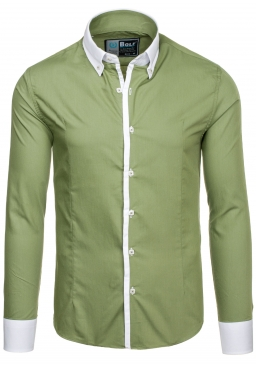# Expensive 17743

The street vendor offered 60 shirts of two types at a total price of CZK 27,300. Cheaper shirts cost 420 CZK, more expensive 480 CZK. How many were there?

a =  25
b =  35

### Step-by-step explanation:

a+b=60
27300 = 420a+480b

a+b=60
27300 = 420·a+480·b

a+b = 60
420a+480b = 27300

Pivot: Row 1 ↔ Row 2
420a+480b = 27300
a+b = 60

Row 2 - 1/420 · Row 1 → Row 2
420a+480b = 27300
-0.14b = -5

b = -5/-0.14285714 = 35
a = 27300-480b/420 = 27300-480 · 35/420 = 25

a = 25
b = 35

Our linear equations calculator calculates it.Did you find an error or inaccuracy? Feel free to write us. Thank you!

Tips for related online calculators
Do you have a linear equation or system of equations and looking for its solution? Or do you have a quadratic equation?